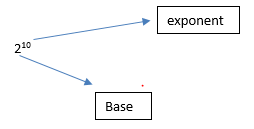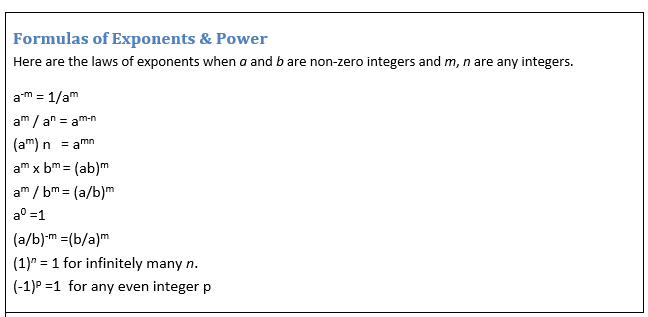# Exponents and powers class 8 notes

In this page we will explain the topics for the chapter 12 of Exponents and Power Class 8 Maths.We have given quality Exponents and powers class 8 notes along with video to explain various things so that students can benefits from it and learn maths in a fun and easy manner, Hope you like them and do not forget to like , social share and comment at the end of the page.
Table of Content

## What is exponent and Base?

This can be explained with the below exampleWe know
22 = 2×2
33 = 3×3×3
So it is known how we interact with positive exponent

## Negative exponents

Here we will be looking how to interact with these type of expression
2-10
11-1
Here we can see that exponents are negative
Now negative exponents can be converted into positive exponents like this
2-10 = 1/210
11-1= 1/11
So
we can say that for any non-zero integer a,
a-m = 1/am
where m is a positive integer. am    is the multiplicative inverse of a-m

Example
a) 2-3
b) 3-2
Solution
As a-m = 1/am
a) 2-3 = ½3 = 1/8
b) 3-2 = 1/32 =1/9

## How to express the decimal number in exponent form

We already know that any non -decimal number can be expressed in exponent form like below
1215 =1000+ 200 +10 +5 = 1 × 103 + 2 × 102 + 2 × 101 + 5 × 100
So these are all positive exponents
Decimal number can be expressed as exponents also
1215.15 =1000+ 2-00 +10 +5 +.10+.05=  1 × 103 + 2 × 102 + 2 × 101 + 5 × 100 +1/10 + 5/100
=1 × 103 + 2 × 102 + 2 × 101 + 5 × 100 +10-1 + 5 ×10-2
So this has both the negative and positive exponents.

## Laws of Exponents

Here are the laws of exponents when a and b are non-zero integers and m, n are any integers.
a-m = 1/am
am / an = am-n
(am )n     = amn
am x bm  = (ab)m
am / bm   = (a/b)m
a0 =1
(a/b)-m =(b/a)m
(1)n = 1 for infinitely many n.
(-1)p =1  for any even integer p
These laws can be used to solve the exponents problems
Example:
(-3)3 × (2/3)3
Solution
(-3)3 × (2/3)3
=(-1)3 × (3)3  × (2)3  × (3)-3   as    am x bm     = (ab)m   and  am / bm       = (a/b)m
= (-1)3 × (2)3
=-8
Important Note
an = 1 only if n = 0. This will work for any a except a = 1 or a = –1. For a = 1, 11 = 12 =13 = 1 or (1)n = 1 for infinitely many n.
For a = –1,(–1)0 = (–1)2 = (–1)4 = (–1)-2 = ... = 1 or (–1)p = 1 for any even integer p.

Watch this tutorial for more explanation About exponents and power

## Use of Exponents to Express Small Numbers in Standard Form

In previous classes we have learnt how to convert large number into exponents form. We can express small number in the standard exponent form also
Let’s take an example to explain
.00001
Step 1:  Count of Number of places after decimal
Here it is 5
Step 2: On the basis of counting in step 1,the number can be written as
.00001 = 1/100000=1/10 = 1X 10-5
as am = 1/a-m
Basically the decimal has been moved to the place after digit
Another example would be
.00019
Step 1:  In these cases, we count of Number of places after decimal to the first digit only
Here it is 4
Step 2: On the basis of counting in step 1,the number can be written as
.0001 = 1.9/10000=1.9/10 = 1.9X 10-4
as am = 1/a-m
Watch this tutorial for more explanation About Use of Exponents to Express Small Numbers in Standard Form

Practice Questions
(a) .00156
(b) .005402
(c) .1234

## Summary

Here is the Exponents and Power Class 8 Notes Summary